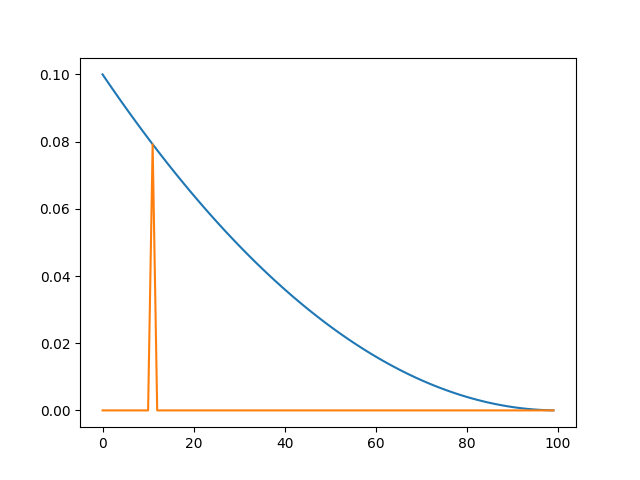# What is the proper way of resuming a scheduler?

PyTorch Version: 1.11
I want to resume my learning rate after my training is terminated.
Here’s a toy example:

``````import torch.nn as nn
from torch.optim import SGD
from torch.optim.lr_scheduler import CosineAnnealingLR

n = 100
net = nn.Conv2d(3, 3, 1)
opt = SGD(net.parameters(), 0.1)
s = CosineAnnealingLR(opt, n, last_epoch=-1)
ckpt = "s.pt"

s_lr = []
for _ in range(s.last_epoch, n):
lr = s.get_last_lr()
s_lr.extend(lr)
s.step()
if _ == 10:
torch.save(s.state_dict(), ckpt)
``````

Now I resume it by two ways. Note that my program is terminated. All object should be instanced again.

``````# Method 1
n = 100
net = nn.Conv2d(3, 3, 1)
opt = SGD(net.parameters(), 0.1)
ckpt = "s.pt"
s = CosineAnnealingLR(opt, n, last_epoch=state_dict["last_epoch"])
s_lr = []
for _ in range(s.last_epoch, n):
lr = s.get_last_lr()
s_lr.extend(lr)
s.step()
print(s_lr[:3])
``````

It throws an error `param 'initial_lr' is not specified in param_groups when resuming an optimizer`. And

``````# Method 2
n = 100
net = nn.Conv2d(3, 3, 1)
opt = SGD(net.parameters(), 0.1)
ckpt = "s.pt"
s = CosineAnnealingLR(opt, n, last_epoch=-1)
s_lr = []
for _ in range(s.last_epoch, n):
lr = s.get_last_lr()
s_lr.extend(lr)
s.step()
print(s_lr[:3])
``````

It print a wrong learning rate `[0.09704403844771128, 0.09942787402278414, 0.09880847171860509]`, which `0.099 > 0.097`.

What is the proper way of resuming a scheduler?

NOTE: I have checked What is the proper way of using last_epoch in a lr_scheduler? but it could not solve my problem.

@ptrblck

Does anyone have some suggesstions?

The first approach should work if you also restore the optimizer and resume from the 10th epoch, since you have also stored the `state_dict` of the scheduler in the 10th step:

``````import torch.nn as nn
from torch.optim import SGD
from torch.optim.lr_scheduler import CosineAnnealingLR

n = 100
net = nn.Conv2d(3, 3, 1)
opt = SGD(net.parameters(), 0.1)
s = CosineAnnealingLR(opt, n, last_epoch=-1)
ckpt = "s.pt"

s_lr = []
for _ in range(s.last_epoch, n):
opt.step()
lr = s.get_last_lr()
s_lr.extend(lr)
s.step()
if _ == 10:
torch.save(s.state_dict(), ckpt)

plt.plot(s_lr)
opt_sd = opt.state_dict()

s_lr = np.array(s_lr)

# Method 1
n = 100
net = nn.Conv2d(3, 3, 1)
opt = SGD(net.parameters(), 0.1)
ckpt = "s.pt"
s = CosineAnnealingLR(opt, n, last_epoch=state_dict["last_epoch"]-1)
s_lr_res = []
for _ in range(s.last_epoch, n):
opt.step()
lr = s.get_last_lr()
s_lr_res.extend(lr)
s.step()

s_lr_res = np.array(s_lr_res)
plt.plot(np.arange(100), s_lr)
plt.plot(np.arange(100), np.concatenate((np.zeros(11), s_lr_res)))

print(s_lr[11:] - s_lr_res)
# [0. 0. 0. 0. 0. 0. 0. 0. 0. 0. 0. 0. 0. 0. 0. 0. 0. 0. 0. 0. 0. 0. 0. 0.
#  0. 0. 0. 0. 0. 0. 0. 0. 0. 0. 0. 0. 0. 0. 0. 0. 0. 0. 0. 0. 0. 0. 0. 0.
#  0. 0. 0. 0. 0. 0. 0. 0. 0. 0. 0. 0. 0. 0. 0. 0. 0. 0. 0. 0. 0. 0. 0. 0.
#  0. 0. 0. 0. 0. 0. 0. 0. 0. 0. 0. 0. 0. 0. 0. 0. 0.]
``````

Output plot:Hi @ptrblck ,

I can reproduce your code and see the same plot using CosineAnnealingLR. But when I tried with PolynomialLR and setting its power=2.0, the plot is strange: the resuming lr curve did not overlap on the old curve, as the attached plot shows. When I enter the debug mode in pycharm, I observed that the resuming scheduler’s last epoch changed to 0 after the first step().What could be the reason for this?

Following is the code I used, I just copied your code snippet and replace the CosineAnnealingLR with PolynomialLR and set its “power” to 2.0.

``````import torch
import torch.nn as nn
from torch.optim import SGD
from torch.optim.lr_scheduler import CosineAnnealingLR
from torch.optim.lr_scheduler import PolynomialLR
import matplotlib.pyplot as plt
import numpy as np

n = 100
net = nn.Conv2d(3, 3, 1)
opt = SGD(net.parameters(), 0.1)
s = PolynomialLR(opt, n, last_epoch=-1, power=2.0)
ckpt = "s.pt"

s_lr = []
for _ in range(s.last_epoch, n):
opt.step()
lr = s.get_last_lr()
s_lr.extend(lr)
s.step()
if _ == 10:
torch.save(s.state_dict(), ckpt)

plt.plot(s_lr)
plt.show()
opt_sd = opt.state_dict()

s_lr = np.array(s_lr)

# Method 1
n = 100
net = nn.Conv2d(3, 3, 1)
opt = SGD(net.parameters(), 0.1)
ckpt = "s.pt"
s = PolynomialLR(opt, n, last_epoch=state_dict["last_epoch"]-1, power=2.0)
s_lr_res = []
for _ in range(s.last_epoch, n):
opt.step()
lr = s.get_last_lr()
s_lr_res.extend(lr)
s.step()

s_lr_res = np.array(s_lr_res)
plt.plot(np.arange(100), s_lr)
plt.plot(np.arange(100), np.concatenate((np.zeros(11), s_lr_res)))
plt.show()
print(s_lr[11:] - s_lr_res)
``````